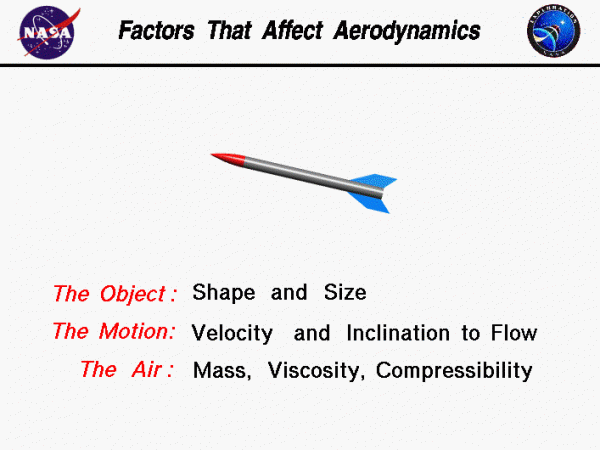# Factors That Affect Aerodynamics## Factors that Affect Aerodynamic Forces

When a solid body is moved through a fluid (gas or liquid), the fluid resists the motion. The object is subjected to an aerodynamic force in a direction opposed to the motion which we call drag. There are many factors that affect aerodynamic forces. We can group these factors into (a) those associated with the object, (b) those associated with the motion of the object through the air, and (c) those associated with the air itself.

## The Object

Geometry has a large effect on the aerodynamic forces generated by an object. Lift and drag depend linearly on the size of the object moving through the air. The cross-sectional shape of an object determines the form drag created by the pressure variation around the object. If we think of drag as aerodynamic friction, the amount of drag depends on the surface roughness of the object; a smooth, waxed surface produces less drag than a roughened surface. This effect is called skin friction and is usually included in the measured drag coefficient of the object.

## Motion of the Air

Lift and drag are associated with the movement of the rocket through the air, so lift and drag depend on the velocity of the air. Lift and drag actually vary with the square of the relative velocity between the object and the air. The inclination of the object to the flow also affects the amount of lift and drag generated by a given shaped object. If the object moves through the air at speeds near the speed of sound, shock waves are formed on the object which create an additional drag component called wave drag. The motion of the object through the air also causes boundary layers to form on the object. A boundary layer is a region of very low speed flow near the surface which contributes to the skin friction.

## Properties of the Air

Lift and drag depend directly on the mass of the flow going past the rocket. The drag also depends in a complex way on two other properties of the air: its viscosity and its compressibility. These factors affect the wave drag and skin friction which are described above.

We can gather all of this information on the factors that affect lift and drag into two mathematical equations called the Lift Equation and the Drag Equation. With the these equations we can predict how much aerodynamic force is generated by a given body moving at a given speed through a given fluid.

Provide feedback